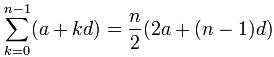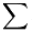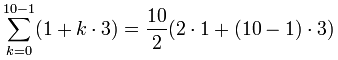# 等差数列及它的和

## 数列## 等差数列

### 例子：

 1，4，7，10，13，16，19，22，25……

{a, a+d, a+2d, a+3d, ... }

• a 是首项，
• d 是项与项之间的差（叫"公差"

### 例子：（续）

 1，4，7，10，13，16，19，22，25……

• a = 1 （首项）
• d = 3 （项与项之间的 "公差"）

{a，a+d，a+2d，a+3d……}

{1，1+3，+2×3，1+3×3……}

{1，4，7，10……}

### 规则

xn = a + d(n-1)

（用 "n-1"，因为在第一项里没有 d

### 例子：写下以下数列的规则，并求其第四项。

 3，8，13，18，23，28，33，38……

• a = 3 （首项）
• d = 5 (（"公差"）

xn = a + d(n-1)

= 3 + 5(n-1)

= 3 + 5n - 5

= 5n - 2

x4 = 5×4 - 2 = 18

## 把等差数列加起来

a + (a+d) + (a+2d) + (a+3d) + ...意思是 "加起来"### 例子：把以下的等差数列的头 10 项加起来：

{ 1，4，7，10，13…… }

• a = 1 （首项）
• d = 3 （"公差"）
• n = 10 （相加多少项）= 5(2+9·3) = 5(29) = 145

## 为什么公式是这样的？

S = a + (a + d) + ... + (a + (n-2)d) + (a + (n-1)d)

S = (a + (n-1)d) + (a + (n-2)d) + ... + (a + d) + a

 S = a + (a+d) + ... + (a + (n-2)d) + (a + (n-1)d) S = (a + (n-1)d) + (a + (n-2)d) + ... + (a + d) + a 2S = (2a + (n-1)d) + (2a + (n-1)d) + ... + (2a + (n-1)d) + (2a + (n-1)d)

2S = n × (2a + (n-1)d)

S = (n/2) × (2a + (n-1)d)